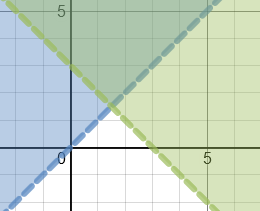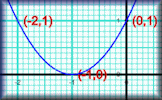# Graph Plotter :: An Online Graphing Calculator

### Ideas for activities

Can you draw pictures with graphs?Model real life situations by translating them into algebraic equations and by using graphs.Find approximate solutions of simultaneous linear equations using graphs. For example

y = 5x - 7

3x + 2y = 1

Plot families of exponential and reciprocal graphs. For example

y = 2x, y = 3x, y = 4x ...

y = 1÷x, y = 2÷x, y = 3÷x, ...

Reduce a given linear equation in two variables to the standard form y = mx + c; calculate gradients and intercepts of the graphs and then plot them to check.

Solve problems involving direct and inverse proportion using graphical representations.Identify and interpret roots, intercepts and turning points of quadratic functions graphically.Find the equations of the translations and reflections of graphs of given functions.Use graphs to solve optimisation problems.Investigate the absolute value function. Plot families of graphs and describe their characteristics. For example

y = |x2 - 5|

Graph inequalities to solve linear programming problems. more...Plot the graphs of functions and their inverses by interchanging the roles of x and y. Find the relationship between the graph of a function and its inverse. Which functions inverses are also functions?

### Related activities

#### Straight Line Graphs10 straight line graph challenges for use with computer graph plotting software or a graphical display calculator.

The short web address is:

Transum.org/go/?to=straight

#### Graph MatchMatch the equations with the images of the corresponding graphs. Linear, quadratic and more.

The short web address is:

Transum.org/go/?to=graphmatch

#### Human GraphsStudents should be encouraged to stand up and make the shapes of the graphs with their arms.

The short web address is:

Transum.org/go/?to=humangraphs

#### Plotting GraphsComplete a table of values then plot the corresponding points to create a linear, quadratic or reciprocal graph.

The short web address is:

Transum.org/go/?to=plotting

The Batman Equation - Turn Anything into a Graph - Sen Zen

Tuesday, October 24, 2017

Tom Lucas, Bristol

Wednesday, February 21, 2018

"It would be nice to be able to draw lines between the table points in the Graph Plotter rather than just the points."

Emmitt, Wesley College

Monday, July 22, 2019

"Would be great if we could adjust the graph via grabbing it and placing it where we want too. thus adjusting the coordinates and the equation."